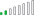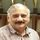cancel
Showing results for
Did you mean:Resolver II

## YTD all employees

Good afternoon,

I have a formula to retrieving the count of employees entering the company for YTD. The formula works in most instances with the exception of a month when there's no type of employees (trans in, new hire, contractor).  How can I retrieve and show the last available count within that month even if the type of employee is blank?

The formula is as followed:

```04_YTD_IN =
Var MaxMonth = IF(SELECTEDVALUE('Date'[Date].[Month]) <> BLANK(), SELECTEDVALUE('Date'[Date].[MonthNo]), MAX('Date'[Date].[MonthNo]))
var MaxYear = FORMAT(MAX('Date'[Date]), "YYYY")
var c_Formula = CALCULATE(SUM(HC[Core HC]), HC[EMP_IN] <> BLANK(), ALL('Date'), HC[YearNum] = MaxYear, VALUE(HC[MonthNum]) <= MaxMonth)
return
c_Formula```

I have a date table with a relationship to my fact table called HC that counts the number of employees coming in from column EMP_IN.

In the image below, the count is 49 for April but I'm only seeing a total of 42.In the next image below, the count is 69 for May and it's populated correctly when all the fields are present.So, the question is, how do I get 7 New Contractors from March to appear in April when it's blank? Thanks for the help!!!

1 ACCEPTED SOLUTIONResolver II

Realizing that the default YTD and custom YTD does not return all cumulative data within the date range I learned that switching over to DATESBETWEEN and ALL did the trick. For those wondering on the solution I came up with, here it is:

```04_YTD_IN =
var MaxYear = MAX(HC[YearNum])
var end_Date = DATEVALUE(SELECTEDVALUE('Date'[Date].[MonthNo]) & "/1/" & MAX('Date'[Date].[Year]))
var MinDate = CALCULATE(FIRSTDATE('Date'[Date]), ALL('Date'), HC[YearNum] = MaxYear)
return
CALCULATE(SUM(HC[Core HC]), FILTER(ALL(HC[EMP_IN]), HC[EMP_IN] <> BLANK()), ALL('Date'), DATESBETWEEN('Date'[Date], MinDate, end_Date))```
2 REPLIES 2Community Champion

Can you please share some data or pbix file to explore a solution.

Cheers

CheenuSing

Did I answer your question? Mark my post as a solution and also give KUDOS !

Proud to be a Datanaut!Resolver II

Realizing that the default YTD and custom YTD does not return all cumulative data within the date range I learned that switching over to DATESBETWEEN and ALL did the trick. For those wondering on the solution I came up with, here it is:

```04_YTD_IN =
var MaxYear = MAX(HC[YearNum])
var end_Date = DATEVALUE(SELECTEDVALUE('Date'[Date].[MonthNo]) & "/1/" & MAX('Date'[Date].[Year]))
var MinDate = CALCULATE(FIRSTDATE('Date'[Date]), ALL('Date'), HC[YearNum] = MaxYear)
return
CALCULATE(SUM(HC[Core HC]), FILTER(ALL(HC[EMP_IN]), HC[EMP_IN] <> BLANK()), ALL('Date'), DATESBETWEEN('Date'[Date], MinDate, end_Date))```